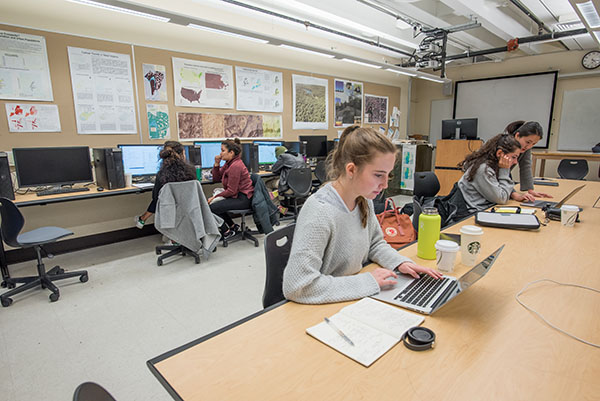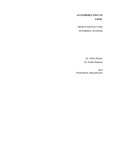## Open Educational Resources: Textbooks

#### Files

Chapter 2: Categorical Propositions (160 KB)

Chapter 3: Categorical Inferences (1.3 MB)

Chapter 4 & 5: Truth Functional Logic, Applications of Modern Logic (861 KB)

Chapter 6: Inductive Logic (184 KB)

Chapter 7: Informal Fallacies (157 KB)

Appendix: Natural Deduction (106 KB)

#### Description

An introduction to the discipline of logic covering subjects from the structures of arguments, classical and modern logic, categorical and inductive inferences, to informal fallacies.

• Over 30 years of development provides a sound empirical based pedagogy throughout the text.
• Examples in ordinary language using familiar examples avoids the suggestion of an alien cultural imposition.
• A focus on the basic representational techniques of classical and modern logic.
• Students introduced to basic concepts of set theory, using Venn diagrams to represent statements and evaluate arguments.
• Students introduced to basic concepts of propositional logic and the use of truth-tables.
• Students introduced to basic concepts of predicate logic and the use of mixed quantifiers.
• Students introduced to the relationship between logic diagrams, circuit diagrams, and gate diagrams in computer science.
• Students introduced to the use of logic in ordinary and scientific contexts.
• Students provided a historical introduction to the development of modern probability theory and its relationship to logic.
• Students introduced to basic concepts of statistical inference, with non-technical treatments of hasty and biased statistical generalizations. And a unique treatment of stereotypical thinking in terms of statistical syllogisms.
• Students introduced to basic notions in analogical and causal inference.
• Exercises requiring both passive (recognition) and active (construction) skills.
• Exercises including locutions and examples from standard English and ethnic dialects of English (African-American, Hispanic-American, etc)
• Answers for sample exercises provided, making the text closer to a self-teaching module

2019

Northampton

#### Keywords

logic, philosophy, OER, textbooks

#### Disciplines

Logic and Foundations of Mathematics | Philosophy